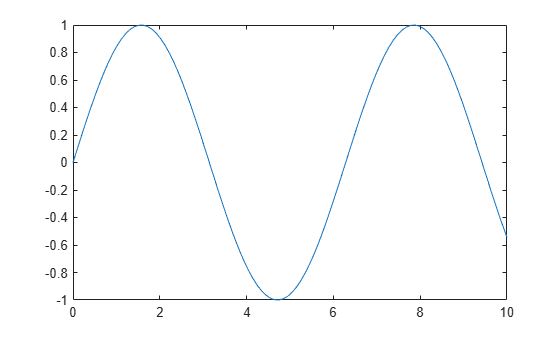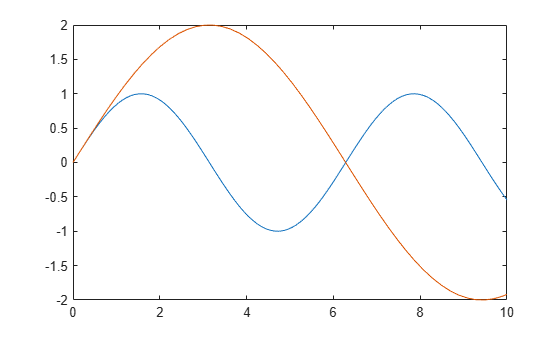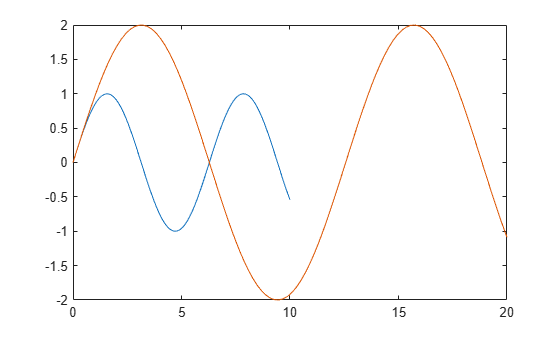Documentation

xlim

Set or query x-axis limits

Description

example

xlim(limits) sets the x-axis limits for the current axes or chart. Specify limits as a two-element vector of the form [xmin xmax], where xmax is greater than xmin.

example

xl = xlim returns the current limits as a two-element vector.

xlim auto sets an automatic mode, enabling the axes to determine the x-axis limits. The limits span the range of the plotted data. Use this option if you change the limits and then want to set them back to the default values. This command sets the XLimMode property for the axes to 'auto'.

example

xlim manual sets a manual mode, freezing the limits at the current values. Use this option if you want to retain the current limits when adding new data to the axes using the hold on command. This command sets the XLimMode property for the axes to 'manual'.

m = xlim('mode') returns the current x-axis limits mode, which is either 'auto' or 'manual'. By default, the mode is automatic unless you specify limits or set the mode to manual.

example

___ = xlim(target,___) uses the axes or chart specified by target instead of the current axes. Specify target as the first input argument for any of the previous syntaxes. You can include an output argument if the original syntax supports an output argument. Use single quotes around the mode inputs, for example, xlim(target,'auto').

Examples

collapse all

Plot a line and set the x-axis limits to range from 0 to 5.

x = linspace(0,10);
y = sin(x);
plot(x,y)
xlim([0 5])Create a surface plot and show only x values greater than 0. Specify the minimum x-axis limit as 0 and let MATLAB choose the maximum limit.

[X,Y,Z] = peaks;
surf(X,Y,Z)
xlim([0 inf])Create a stem chart with dates along the x-axis. Set the x-axis limits to range from June 1, 2014 to June 5, 2014.

t = datetime(2014,06,1) + caldays(0:10);
y = rand(11,1);
stem(t,y,'filled')

tstart = datetime(2014,06,1);
tend = datetime(2014,06,5);
xlim([tstart tend])Starting in R2019b, you can display a tiling of plots using the tiledlayout and nexttile functions. Call the tiledlayout function to create a 2-by-1 tiled chart layout. Call the nexttile function to create the axes objects ax1 and ax2. Plot data into each axes. Then set the x-axis limits for the bottom plot by specifying ax2 as the first input argument to xlim.

tiledlayout(2,1)
x = linspace(0,5,1000);
y = sin(100*x)./exp(x);
ax1 = nexttile;
plot(ax1,x,y)

ax2 = nexttile;
plot(ax2,x,y)
xlim(ax2,[0 1])Use manual mode to maintain the current x-axis limits when you add more plots to the axes.

First, plot a line.

x = linspace(0,10);
y = sin(x);
plot(x,y);Set the x-axis limits mode to manual so that the limits do not change. Use hold on to add a second plot to the axes.

xlim manual
hold on
plot(2*x,2*y)
hold offThe x-axis limits do not update to incorporate the new plot.

Switch back to automatically updated limits by resetting the mode to automatic.

xlim autoCreate a scatter plot of random data. Return the values of the x-axis limits.

x = randn(50,1);
y = randn(50,1);
scatter(x,y)xl = xlim
xl = 1×2

-3     4

Input Arguments

collapse all

Minimum and maximum limits, specified as a two-element vector of the form [xmin xmax], where xmax is greater than xmin. You can specify the limits as numeric, categorical, datetime, or duration values. However, the type of values that you specify must match the type of values along the x-axis.

You can specify both limits, or specify one limit and let MATLAB® automatically calculate the other. For an automatically calculated minimum or maximum limit, use -inf or inf, respectively.

Example: xlim([0 1])

Example: xlim([-inf 1])

Example: xlim([0 inf])

Data Types: single | double | int8 | int16 | int32 | int64 | uint8 | uint16 | uint32 | uint64 | categorical | datetime | duration

Target axes or chart, specified as an Axes object or a graphics object with an XLimits property, such as a HeatmapChart object. If you do not specify the axes or chart, then xlim sets the limits for the current axes or chart (returned by gca).

Output Arguments

collapse all

Current limits, returned as a two-element vector of the form [xmin xmax].

Querying the limits returns the XLim or XLimits property value for corresponding Axes or graphics object.

Current limits mode, returned as one of these values:

• 'auto' — Automatically determine the limits.

• 'manual' — Use manually specified limits that do not update to reflect changes in the data.

Querying the x-axis limits mode returns XLimMode property value for the corresponding Axes object.

Algorithms

The xlim function sets and queries several axes properties related to the x-axis limits.

• XLim — Property that stores the x-axis limits.

• XLimMode — Property that stores the x-axis limits mode. When you set the x-axis limits, this property changes to 'manual'.